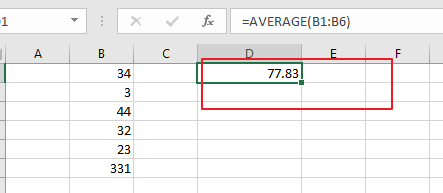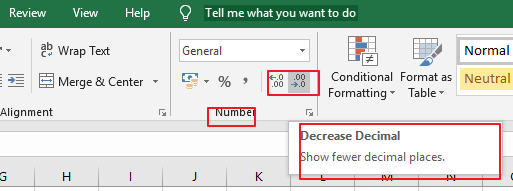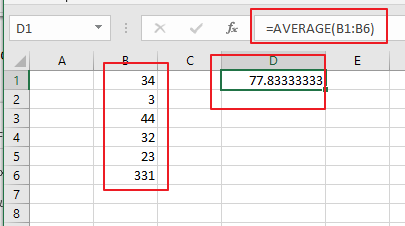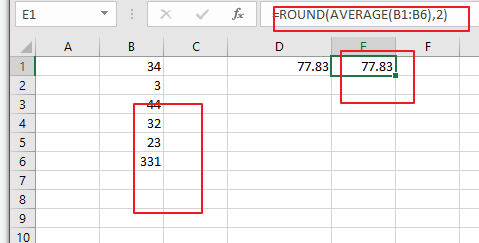# How to Round the Calculated Average result in Excel

This post will guide you how to use average and round functions at the same time to average a given range, and then round off the last result in Excel. How to use Round function to round the calculated average values with a formula in Excel.

Assuming that you have a list of data in range B1:B6 in which contain numeric values. And you can easily to calculate the average value with AVERAGE function. And the average value may contain two or more decimal places. And you want to round off this number to the specified number of decimal places. How to do it. You can use another function called ROUND. This post will show you tow methods to accomplish the result.

## Round the Calculated Average result with Decimal Command

To round the calculated average value for your data, you can use increase or decrease decimal command with the following steps:

Step1: select the range that to be averaged. Such as: B1:B6.

Step2: type the following formula based on the AVERAGE function to calculate the average value for the given range of cells:

`=AVERAGE(B1:B6)`

Type this formula into a blank cell and press Enter key on your keyboard. You would see that the average value has been calculated.Step3: select the calculated average value and go to Home tab, and click Increase decimal button or decrease decimal button under Number group.## Round the Calculated Average result with a Formula

You can also use a formula based on the ROUND function and AVERAGE function to accomplish the same result of rounding the calculated average result in Excel. Like the following formula:

`=ROUND(AVERAGE(B1:B6),2)`

Note: if you want to round off to the specified number of decimal places as you need, you just need to change the number 2 as you want.### Related Functions

• Excel Round function
The Excel INT function rounds a number to a specified number of digits. You can use the ROUND function to round to the left or right of the decimal point in Excel.The syntax of the ROUND function is as below:=ROUND (number, num_digits)…
• Excel AVERAGE function
The Excel AVERAGE function returns the average of the numbers that you provided.The syntax of the AVERAGE function is as below:=AVERAGE (number1,[number2],…)….

Sidebar